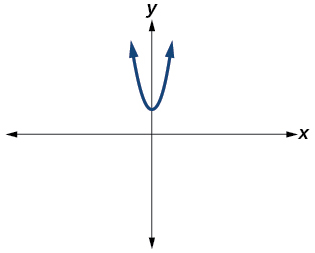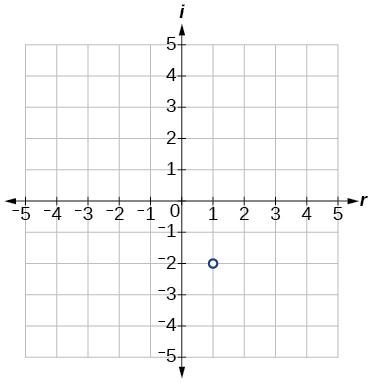# 3.1E: Exercises

$$\newcommand{\vecs}{\overset { \rightharpoonup} {\mathbf{#1}} }$$ $$\newcommand{\vecd}{\overset{-\!-\!\rightharpoonup}{\vphantom{a}\smash {#1}}}$$$$\newcommand{\id}{\mathrm{id}}$$ $$\newcommand{\Span}{\mathrm{span}}$$ $$\newcommand{\kernel}{\mathrm{null}\,}$$ $$\newcommand{\range}{\mathrm{range}\,}$$ $$\newcommand{\RealPart}{\mathrm{Re}}$$ $$\newcommand{\ImaginaryPart}{\mathrm{Im}}$$ $$\newcommand{\Argument}{\mathrm{Arg}}$$ $$\newcommand{\norm}{\| #1 \|}$$ $$\newcommand{\inner}{\langle #1, #2 \rangle}$$ $$\newcommand{\Span}{\mathrm{span}}$$ $$\newcommand{\id}{\mathrm{id}}$$ $$\newcommand{\Span}{\mathrm{span}}$$ $$\newcommand{\kernel}{\mathrm{null}\,}$$ $$\newcommand{\range}{\mathrm{range}\,}$$ $$\newcommand{\RealPart}{\mathrm{Re}}$$ $$\newcommand{\ImaginaryPart}{\mathrm{Im}}$$ $$\newcommand{\Argument}{\mathrm{Arg}}$$ $$\newcommand{\norm}{\| #1 \|}$$ $$\newcommand{\inner}{\langle #1, #2 \rangle}$$ $$\newcommand{\Span}{\mathrm{span}}$$$$\newcommand{\AA}{\unicode[.8,0]{x212B}}$$

## Verbal

Exercise $$\PageIndex{1}$$

Explain how to add complex numbers.

Add the real parts together and the imaginary parts together.

Exercise $$\PageIndex{2}$$

What is the basic principle in multiplication of complex numbers?

Exercise $$\PageIndex{3}$$

Give an example to show the product of two imaginary numbers is not always imaginary.

$$i$$ times $$i$$ equals –1, which is not imaginary. (answers will vary)

Exercise $$\PageIndex{4}$$

What is a characteristic of the plot of a real number in the complex plane?

## Algebraic

For the following exercises, evaluate the algebraic expressions.

Exercise $$\PageIndex{5}$$

If $$f(x)=x^2+x−4$$, evaluate $$f(2i)$$.

$$−8+2i$$

Exercise $$\PageIndex{6}$$

If $$f(x)=x^3−2$$, evaluate $$f(i)$$.

Exercise $$\PageIndex{7}$$

If $$f(x)=x^2+3x+5$$,evaluate $$f(2+i)$$.

$$14+7i$$

Exercise $$\PageIndex{8}$$

If $$f(x)=2x^2+x−3$$, evaluate $$f(2−3i)$$.

Exercise $$\PageIndex{9}$$

If $$f(x)=\frac{x+1}{2−x}$$, evaluate $$f(5i)$$.

$$−\frac{23}{29}+\frac{15}{29}i$$

Exercise $$\PageIndex{10}$$

If $$f(x)=\frac{1+2x}{x+3}$$, evaluate $$f(4i)$$.

## Graphical

For the following exercises, determine the number of real and nonreal solutions for each quadratic function shown.

Exercise $$\PageIndex{11}$$2 real and 0 nonreal

Exercise $$\PageIndex{12}$$For the following exercises, plot the complex numbers on the complex plane.

Exercise $$\PageIndex{13}$$

$$1−2i$$Exercise $$\PageIndex{14}$$

$$−2+3i$$

Exercise $$\PageIndex{15}$$

$$i$$Exercise $$\PageIndex{16}$$

$$−3−4i$$

## Numeric

For the following exercises, perform the indicated operation and express the result as a simplified complex number.

Exercise $$\PageIndex{17}$$

$$(3+2i)+(5−3i)$$

$$8−i$$

Exercise $$\PageIndex{18}$$

$$(−2−4i)+(1+6i)$$

Exercise $$\PageIndex{19}$$

$$(−5+3i)−(6−i)$$

$$−11+4i$$

Exercise $$\PageIndex{20}$$

$$(2−3i)−(3+2i)$$

Exercise $$\PageIndex{21}$$

$$(−4+4i)−(−6+9i)$$

$$2−5i$$

Exercise $$\PageIndex{22}$$

$$(2+3i)(4i)$$

Exercise $$\PageIndex{23}$$

$$(5−2i)(3i)$$

$$6+15i$$

Exercise $$\PageIndex{24}$$

$$(6−2i)(5)$$

Exercise $$\PageIndex{25}$$

$$(−2+4i)(8)$$

$$−16+32i$$

Exercise $$\PageIndex{26}$$

$$(2+3i)(4−i)$$

Exercise $$\PageIndex{27}$$

$$(−1+2i)(−2+3i)$$

$$−4−7i$$

Exercise $$\PageIndex{28}$$

$$(4−2i)(4+2i)$$

Exercise $$\PageIndex{29}$$

$$(3+4i)(3−4i)$$

25

Exercise $$\PageIndex{30}$$

$$\frac{3+4i}{2}$$

Exercise $$\PageIndex{31}$$

$$\frac{6−2i}{3}$$

$$2−\frac{2}{3}i$$

Exercise $$\PageIndex{32}$$

$$\frac{−5+3i}{2i}$$

Exercise $$\PageIndex{33}$$

$$\frac{6+4i}{i}$$

$$4−6i$$

Exercise $$\PageIndex{34}$$

$$\frac{2−3i}{4+3i}$$

Exercise $$\PageIndex{35}$$

$$\frac{3+4i}{2−i}$$

$$\frac{2}{5}+\frac{11}{5}i$$

Exercise $$\PageIndex{36}$$

$$\frac{2+3i}{2−3i}$$

Exercise $$\PageIndex{37}$$

$$\sqrt{−9}+3\sqrt{−16}$$

$$15i$$

Exercise $$\PageIndex{38}$$

$$−\sqrt{−4}−4\sqrt{−25}$$

Exercise $$\PageIndex{39}$$

$$\frac{2+\sqrt{−12}}{2}$$

$$1+i\sqrt{3}$$

Exercise $$\PageIndex{40}$$

$$\frac{4+\sqrt{−20}}{2}$$

Exercise $$\PageIndex{41}$$

$$i^8$$

$$1$$

Exercise $$\PageIndex{42}$$

$$i^{15}$$

Exercise $$\PageIndex{43}$$

$$i^{22}$$

$$−1$$

## Technology

For the following exercises, use a calculator to help answer the questions.

44. Evaluate $$(1+i)^k$$ for $$k=4, 8,$$ and $$12$$.Predict the value if $$k=16$$.

45. Evaluate $$(1−i)^k$$ for $$k=2, 6,$$ and $$10$$.Predict the value if $$k=14$$.

46. Evaluate (1+i)k−(1−i)k for $$k=4$$, 8, and 12. Predict the value for $$k=16$$.

47. Show that a solution of $$x^6+1=0$$ is $$\frac{\sqrt{3}}{2}+\frac{1}{2}i$$.

Answer: $$(\frac{\sqrt{3}}{2}+\frac{1}{2}i)^6=−1$$

48. Show that a solution of $$x^8−1=0$$ is $$\frac{\sqrt{2}}{2}+\frac{\sqrt{2}}{2}i$$.

## Extensions

For the following exercises, evaluate the expressions, writing the result as a simplified complex number.

49. $$\frac{1}{i}+\frac{4}{i^3}$$

Answer: $$3i$$

50. $$\frac{1}{i^{11}}−\frac{1}{i^{21}}$$

51. $$i^7(1+i^2)$$

52. $$i^{−3}+5i^7$$

53. $$\frac{(2+i)(4−2i)}{(1+i)}$$

Answer: $$5 – 5i$$

54. $$\frac{(1+3i)(2−4i)}{(1+2i)}$$

55. $$\frac{(3+i)^2}{(1+2i)^2}$$

Answer: $$−2i$$

56. $$\frac{3+2i}{2+i}+(4+3i)$$

57. $$\frac{4+i}{i}+\frac{3−4i}{1−i}$$

Answer: $$\frac{9}{2}−\frac{9}{2}i$$

58. $$\frac{3+2i}{1+2i}−\frac{2−3i}{3+i}$$

3.1E: Exercises is shared under a CC BY-NC-SA license and was authored, remixed, and/or curated by LibreTexts.